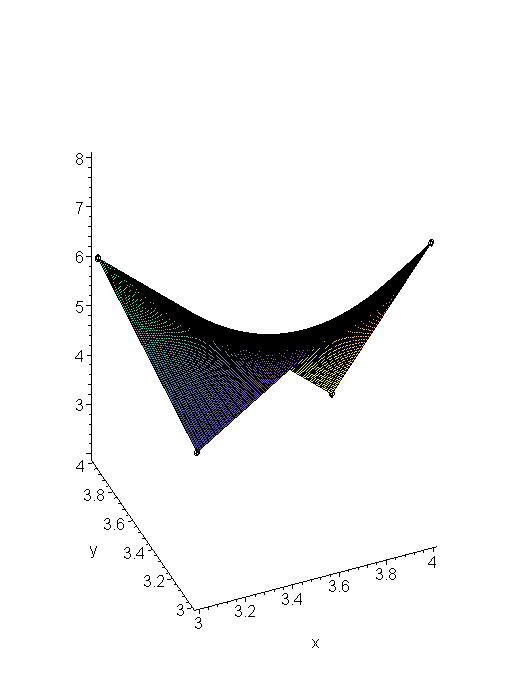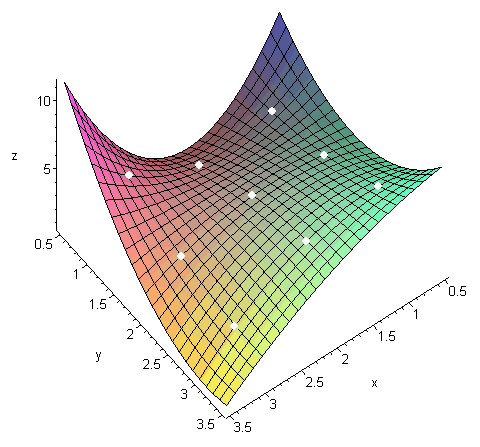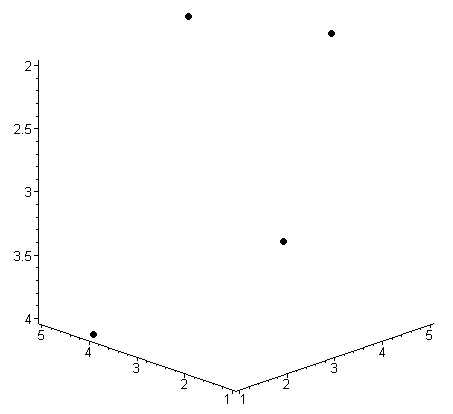# Introduction

We can generalize the Vandermonde method to interpolate multivariate real-valued functions. We will focus on bivariate polynomials, and the generalization is obvious. For example, we may have a surface defined by a function of x and y, e.g., f(x, y). Given points ((x1, y1), z1), ((x2, y2), z2), ..., ((xn, yn), zn) find an interpolating polynomial. By simply substituting the points into the bivariate polynomial, you naturally get a system of linear equations in the coefficients which may then be solved using Gaussian elimination or LU decomposition.

This technique also generalizes for interpolating non-polynomial functions.

# Background

Useful background for this topic includes:

• None.

# The Vandermonde Method

Suppose we have 4 2D points (0, 1), (2, 5), (4, 3), and (6, 7) and we have values for each of these points, e.g., ((0, 1), 13), ((2, 5), 17), ((4, 3), 15), and ((6, 7), 18) and we want to fit a bivariate polynomial through these points.

From the Vandermonde method, it would make sense that we could find an interpolating bivariate polynomial with four terms, for example:

p(x) = c1 xy + c2 x + c3 y + c4

Thus, if we were to simply evaluate p(x, y) at these four points, we get four equations:

p(0, 1) = c1 0 + c2 0 + c3 1 + c4 = 13
p(2, 5) = c1 10 + c2 2 + c3 5 + c4 = 17
p(4, 3) = c1 12 + c2 4 + c3 3 + c4 = 15
p(6, 7) = c1 42 + c2 6 + c3 7 + c4 = 19

As before, this defines a system of linear equations which we may solve.

## Observation

This method works best with the points form a grid, for example, a sequence of points like: (0, 0), (0, 1), (1, 0), (1, 1). In this case, the Vandermonde matrix is the rather simple

# HOWTO

Find an interpolating polynomial which passes through n points ((x1, y1), z1), ..., ((xn, yn), zn).

# Assumptions

The (x, y) pairs of values are unique.

# Process

Because there are n points, the degree of the interpolating polynomial must have n terms. Thus, the form of the interpolating polynomial may be various, for example, given four points in a square, e.g., (0, 0), (0, 1), (1, 0), (1, 1), the logical choice is:

p(x, y) = c1 xy + c2 x + c3 y + c4.

Given nine points in a square, we could use:

p(x, y) = c1 x2y2 + c2 x2y + c3 xy2 + c4 x2 + c5 y2 + c6 xy + c7 x + c8 y + c9.

Next we define the n × n Vandermonde matrix V by evaluating the n terms at each of the n points. For example, given the first example above (with four points), we would define the matrix

NOTE: the right-hand column is 1s if and only if the last function is the constant function 1.

For example, the Vandermonde matrix for finding the polynomial which interpolates the four points ((2, 2), 12), ((3, 6), 15), ((5, 4), 13), ((7, 7), 18) is:

Note that the Vandermonde matrix is independent of the z values.

The generalization to fitting a sequence of arbitrary functions is obvious.

To be completed.

# Examples

1. Find the polynomial which interpolates the points ((3, 3), -1), ((3, 4), 2), ((5, 3), 1).

Because there are three points, the interpolating polynomial could be of the form p(x, y) = c1x + c2y + c3. Thus, we define the Vandermonde matrix

and solve the system Vc = z where z = (-1, 2, 1)T. This gives the result c = (1, 3, -13)T, and therefore the interpolating polynomial is p(x, y) = x + 3 y − 13.

If x = (x, y)T, then we could write this polynomial as p(x) = x1 + 3 x2 − 13.

2. Find the polynomial which interpolates the points ((3, 3), 5), ((3, 4), 6), ((4, 3), 7), ((4, 4), 9).

Because there are four points, the interpolating polynomial could reasonably be of the form p(x) = c1xy + c2x + c3y + c4. Thus, we define the Vandermonde matrix

and solve the system Vc = z where z = (5, 6, 7, 9)T. This gives the result c = (1, -1, -2, 5)T, and therefore the interpolating polynomial is p(x, y) = xyx − 2 y + 5.

A plot of the four points and the interpolating polynomial is shown in Figure 1.Figure 1. An interpolating bivariate polynomial.

3. Find the interpolating polynomial passing through these nine points: (1, 1, 3.2), (1, 2, 4.4), (1, 3, 6.5), (2, 1, 2.5), (2, 2, 4.7), (2, 3, 5.8), (3, 1, 5.1), (3, 2, 3.6), (3, 3, 2.9).

The following Matlab code represents the x, y, and z values and finds the interpolating polynomial of the form c2,2x2y2 + c2,1x2y + c2,0x2 + c1,2xy2 + c1,1xy + c1,0x + c0,2y2 + c0,1y + c0,0.

```>> x = [1 1 1 2 2 2 3 3 3]';
>> y = [1 2 3 1 2 3 1 2 3]';
>> z = [3.2 4.4 6.5 2.5 4.7 5.8 5.1 3.6 2.9]';
>> V = [x.^2.*y.^2 x.^2.*y x.^2 x.*y.^2 x.*y x y.^2 y x.^0]

V =

1    1    1    1    1    1    1    1    1
4    2    1    4    2    1    4    2    1
9    3    1    9    3    1    9    3    1
4    4    4    2    2    2    1    1    1
16    8    4    8    4    2    4    2    1
36   12    4   18    6    2    9    3    1
9    9    9    3    3    3    1    1    1
36   18    9   12    6    3    4    2    1
81   27    9   27    9    3    9    3    1

>> c = V \ z
c =

0.97500
-5.27500
5.95000
-3.92500
19.82500
-21.55000
3.40000
-14.70000
18.50000
```

A plot of the points and the interpolating polynomial are shown in Figure 2.Figure 2. The interpolating polynomial of nine points.

4. The three above examples have placed the points on a grid; this is not a requirement for multivariate interpolation. The interpolating polynomial of the form p(x,y,z) = c1,0,0x + c0,1,0y + c0,0,1z + c0,0,0 passing through the points (1, 2, 3, 4.9), (3, 5, 2, 2.6), (5, 4, 2, 3.7), (4, 1, 4, 7.8).

```>> x = [1 3 5 4]';
>> y = [2 5 4 1]';
>> z = [3 2 2 4]';
>> w = [4.9 2.6 3.7 7.8]';
>> V = [x y z ones(4,1)]
V =
1   2   3   1
3   5   2   1
5   4   2   1
4   1   4   1

>> c = V \ w
c =
0.31111
-0.47778
1.48889
1.07778
```

While it is difficult to show a function in four dimensions, Figure 3 shows the points and to demonstrate the validity:

0.31111·1 − 0.47778·2 + 1.48889·3 + 1.07778 = 4.9Figure 3. The four points interpolated in Example 4.

# Questions

1. Find the bivariate polynomial which interpolates the points ((0, 0), 5), ((0, 1), 4), ((1, 0), 3), and ((1, 1), 6).

Answer: p(x, y) = 4xy − 2xy + 5.

2. Find the bivariate polynomial which interpolates the points ((2, 4), 12), ((2, 5), 11), ((3, 4), 10), and ((3, 5), 14).

Answer: p(x, y) = 5xy − 22x − 11y + 60.

3. Find the bivariate polynomial which interpolates the points ((2, 2), 6), ((2, 3), 10), ((4, 2), 12), and ((5, 5), 18).

Answer: p(x, y) = -xy + 5x + 6y − 12.

4. Use Matlab to find the bivariate polynomial which interpolates the points ((2, 3), 16), ((2, 5), 15), ((4, 3), 14), and ((6, 6), 17).

Answer: p(x, y) = 0.5417 xy − 2.625 x − 1.5833 y + 22.75.

# Applications to Engineering

Many systems are functions of more than one variable, and interpolating a surface between a set of points may be necessary.

One example is in graphics where one may wish to smoothly zoom in on a pixelated image.

# Matlab

There is no easy way of generating the

```>> x = [1 2 1 2]';
>> y = [1 1 2 2]';
>> z = [5 3 0 4]';
>> V = [x .* y x y ones(size(x))];
>> c = V \ z
c =

6
-8
-11
18
```

# Maple

There are no tools to make multivariate interpolation any easier than what is presented.